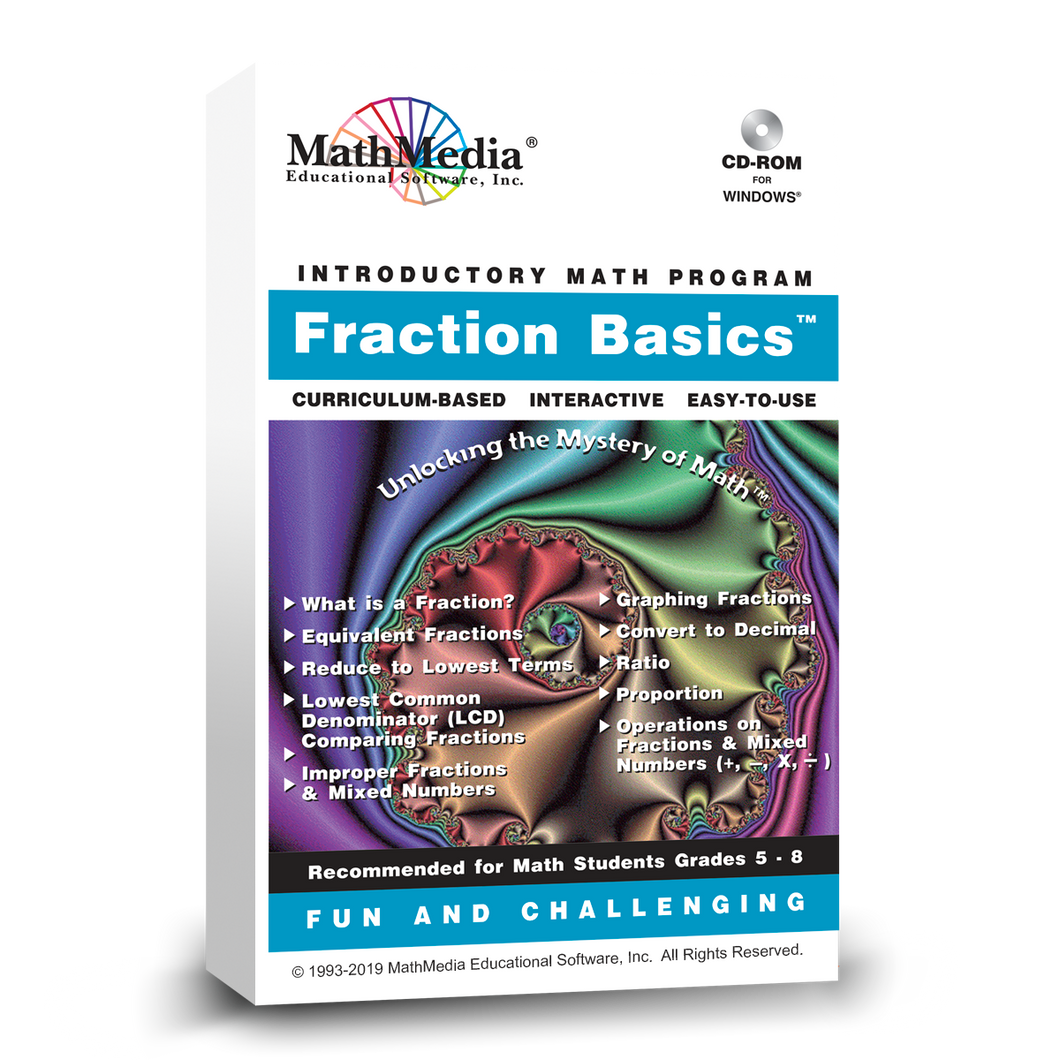# Fraction Basics™

Regular price \$49.00 \$12.25 Sale

This is the 1st math software program in the "Basic Math Series". Learning fractions with this software program is comprehensive, complete, and organized methodically from comparing fractions to adding and subtracting fractions to multiplying fractions. Instructors use this software to aid in teaching fractions. Learn math with this first program in the "Basic Math Series" curriculum in an organized systematic approach.

MENU #1: What is a Fraction?, Equivalent Fractions, Reducing Fractions to Lowest Terms, LCD, Comparing Fractions, Improper Fractions, Mixed Numbers, Graphing Fractions, and Converting a Fraction to a Decimal.

MENU #2: What is a Ratio?, What is a Proportion?, Adding Fractions with the same denominator and Adding Fractions with different denominators, Subtracting Fractions with the same denominator and Subtracting Fractions with different denominators, Multiplying Fractions with a simple method and Multiplying Fractions with the cancellation method, Dividing Fractions, Fractions of Numbers, and Operations on Mixed Numbers. Although these topics in fractions are generally taught in 4th grade math and 5th grade math, they appear throughout the math curriculum. Adult students returning to their math education will benefit from learning math with these math software programs. Improve students' math skills. Suitable for elementary school, high school, or developmental college math students. This math help software provides tips and techniques for elementary math student and teachers who are teaching fractions through high school students. This "Fraction Basics" software program offers one-on-one help for math students using a variety of methods.

TEST: The program concludes with a test which is scored by the computer. A printout of the student's answers and which sections require additional learning is provided.
This material is presented to classroom students over the course of years - some concepts beginning as early as elementary school and continuing through high school. Use this tutorial software to keep up with schoolwork, to learn at home, to remediate, to accelerate, as a course or as a reference.